# Using Bayesian optimization for balancing metrics in recommendation systems

##Yunbo Ouyang <div class="new-miniprofile-content"> <i class="triangle triangle-top top right"></i> <div class="miniprofile-body "> <p class="photo"> <a href="/content/engineering/en-us/blog/authors/y/yunbo-ouyang.html"> <img src="/content/dam/engineering/site-assets/images/blog/authors/y/yunbo-ouyang.jpg" altText="Yunbo Ouyang" /> </a> </p> <h2 class="name"><a href="/content/engineering/en-us/blog/authors/y/yunbo-ouyang.html">Yunbo Ouyang</a></2> <ul class="miniprofile-actions"> <li class="action-item"><a href="/content/engineering/en-us/blog/authors/y/yunbo-ouyang.html" class="miniprofile-button bt-send">View all posts</a></li> <li class="action-item"><a href="https://www.linkedin.com/in/yunbo-ouyang-25159490" target="_blank" class="miniprofile-button bt-secondary">View LinkedIn Profile</a></li> </ul> </div> <i class="triangle triangle-bottom top right"></i> </div> February 4, 2022

Co-authors: Yunbo Ouyang,Viral Gupta, Kinjal Basu, Cyrus Diciccio, Brendan Gavin, and Lin Guo.

Most large-scale recommender systems like newsfeed ranking, people recommendations, job recommendations, etc., have multiple objectives that need to be simultaneously optimized. These objectives may include things like user engagement, diversity, novelty, freshness, or fairness. These objectives can sometimes be conflicting, so there is a need to balance them. Multi-objective optimization (MOO) is used for many products at LinkedIn (such as the homepage feed) to help balance different behaviors in our ecosystem. As we’ve discussed previously, there are two main components to MOO: training models that predict the likelihood of a certain behavior occurring (like applying for a job), and optimization workflows to search for the optimal hyperparameters to balance the different possible objectives.

We’ve previously shared how we automate the tuning of parameters in our MOO machine learning model that recommends content on the newsfeed; in this blog post, we’ll focus on the generic optimization methodology and the unified platform we have built that enables onboarding new use cases easily. An example of a situation where we optimize for multiple objectives is the LinkedIn Notifications recommendation system, which notifies members about various activities within their network. The objective is to improve click-through-rate (CTR) and increase the number of sessions by keeping guardrail metrics like “disables” neutral. The CTR and sessions objectives can be conflicting, because sending more notifications to our members may increase the overall number of sessions, but can decrease CTR because the quality of the notification might drop. Separate models optimize for CTR and sessions, and then a linear combination of the output of those models is used to send notifications to members.

Suppose we have n metrics m1, m2... mn and we built models M1, M2... Mn to optimize for each of them. The final model M is

Mx=M1 + x1*M2 + x2*M3 + xn-1*Mn

where x=(x1 ,x2 xn-1) is a tunable combination parameter vector used to balance different objectives. Different models Mx may form the Pareto Front, so that we cannot optimize one metric without hurting other metrics.

To search for a suitable x, we identify one metric as the primary metric (e.g., m1) and other metrics as guardrail metrics. We conduct online A/B experimentation to launch the model Mx and collect metrics m1(x), m2(x)... mn(x) to find the solution of the following constrained optimization problem:

•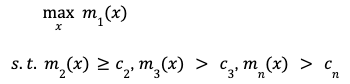c2,cn are threshold values, which are the metrics of the control model in the A/B experiment. Random search and grid search are often used to try different combinations of parameters x.

Launching A/B tests in these situations can be complex because:

1. A/B tests require a large sample size. In LinkedIn’s production environment, there are multiple A/B tests running concurrently. The sample size available to tune the combination parameters is limited.

2. A/B tests are not adaptive to the potential promising variants. We want to repurpose the traffic to more promising options on the fly, which reduces the risk of poor variants running for too long. This requires shifting traffic away from some of the variants that have poor metrics. However, traditional A/B tests cannot achieve this.

In addition, setting up A/B tests can also be time consuming. Manually configuring A/B tests and monitoring them is not the best use of engineers’ time.

## Bayesian optimization

To overcome these challenges, we apply Bayesian optimization to solve

•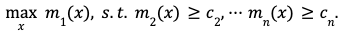Bayesian optimization is a sequential optimization strategy for optimizing expensive-to-evaluate, “opaque-box” functions; it sequentially searches for the optimal hyperparameters until convergence. It is an approach used to model objectives whose functional forms are unknown. In this approach, a surrogate is built for the objective function and the uncertainty in the objective function is quantified using Gaussian process regression. Bayesian optimization consists of two components:

1. A function fitting method to produce posterior distributions of unknown functions;

2. An acquisition function to suggest the next candidate.

Online metrics m1(x), m2(x)... mn(x) are noisy and nonlinear. Gaussian processes are used to model the metrics. We apply the following hierarchical model to model the underlying mean fi(x) of the ith metric mi(x).

•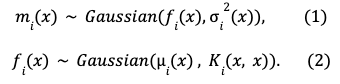𝜎i2(x) is the pre-specified noise level or contains a free parameter to estimate the noise level. The latent function fi(x) follows Gaussian distribution with prior mean function 𝜇i(x) and prior covariance function Ki(x, x). 𝜇i(x) is usually a constant. We refer to Ki(x1, x2) as a kernel function to map x1, x2 to the covariance between fi(x1) and fi(x2). Kernel functions contain unknown hyperparameters to be optimized by maximizing the marginal likelihood of mi(x). Popular choices for kernels include RBF kernel and Matérn kernel. After kernel hyperparameters are replaced by the estimated values, the Gaussian process produces posterior distributions for fi(x). Since the metric has an intrinsic noise (see (1) and (2) in the equations above), the goal is to write the optimization problem formulation to maximize the underlying mean function. The above optimization problem can be written as

•Then, we convert the constraint optimization problem into an unconstrained optimization problem by converting constraints into indicator functions:

•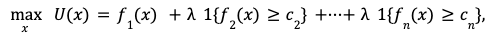where ƛ is a large positive constant and 1{fi(x)≥ci} is an indicator function. When fi(x)≥ci, ƛ is contributed to the total utility U(x). Since we have obtained posterior distributions of f1(x), f2(x),ॱ ॱ ॱ fn(x), we can easily obtain the posterior distribution of the total utility U(x).

The second component of Bayesian optimization is to define an acquisition function to suggest the next candidate. We choose Thompson sampling because it provides probabilistic suggestions. Suppose we have N candidates x1, x2,ॱ ॱ ॱ ,xN. Thompson sampling suggests that with probability pi=P(U(xi)  is the largest among U(x1),ॱ ॱ ॱ , U(xN)), we choose xi. If the probability of xi being optimal does not have a closed form, we can use Monte Carlo sampling to approximate it—that is, we draw a large number of posterior samples from U(x) and count the frequency of each xi being optimal.

The output for one iteration of Bayesian optimization is discrete distribution Ft that can be represented as a list of tuples (𝒙1, p1),ॱ ॱ ॱ ,(𝒙N, pN). This output naturally aligns with the online A/B test framework: we randomly split the treatment group into N groups, each group has pi percent subjects, and they are served with the model using the combination parameter xi

In the next section, we will demonstrate how we apply Bayesian optimization to find optimal hyperparameters for tuning Notification models.

•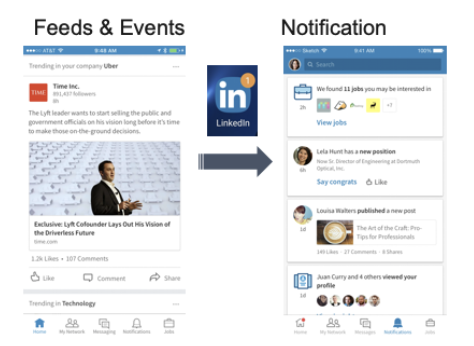In the LinkedIn app, Notifications and emails serve as an important channel to update members about an activity that they may have missed. For example, in Figure 1 we see there is an article shared by the Lyft co-founder talking about his vision of a driverless future. This can be a good notification candidate for members who are interested in the self-driving space. Such notifications fall in the category of activity-based notifications.

The Notifications platform is a streaming system that reads the activity events from a Kafka queue. Each activity event that is created has an associated content identifier (id) and an actor id. Given the content id ck, actor id ai, we generate candidates to provide the set of n recipients rikj, 1≤j≤n who may be interested in being notified. For each tuple {ai, ck, rikj}, we want to appropriately send or drop the notification. The goal of sending a notification is to connect members with content they find engaging. There are several ways to measure the efficacy of sending activity-based notifications. The most obvious is the clicks on the notifications that are sent to the members. Notifications may also spur members to visit the platform to start a session. We model both these aspects through separate ML models.

For a notification candidate, the two models’ scores are combined as shown below, and if the score is above the threshold 𝛾, the notification gets sent.

•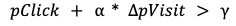Where

• pClick models the probability of a click by member rijk.
• ΔpVisit is the difference in probability of a visit (during a fixed time horizon) between sending a notification now versus not sending a notification. It can also be written as p(Visit | send ) - p(Visit | not send).

• a is the hyperparameter that measures the relative importance of the two utilities.

• 𝛾 is the threshold applied.

For ramping the models, we want to find x={a,𝛾}, and we look at business metrics like Sessions, Impressed CTR, and Send Volume of Notifications. See definitions below:

We solve the following optimization problem:

•We want to find the value of x={a,𝛾} such that we can maximize the Sessions for the treatment model while honoring constraints on Impressed CTR and Send Volume. c1 and c2 are constants to ensure the new model performs reasonably well compared to an existing control model.

The above problem can be solved by applying Bayesian optimization as described in the previous section. First, we use a Gaussian process to fit Sessions(x), Impressed CTR(x), and Send Volume(x). Then, we use the fitted function to replace the original metrics with fitted functions.

•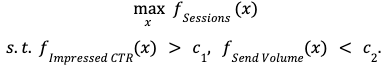Finally, we define a single function to optimize:

•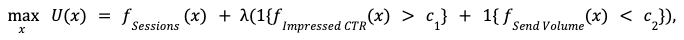ƛ is a large constant to guarantee all constraints are satisfied. Thompson sampling is applied to obtain the discrete distribution Ft, that is represented as a list of (combination parameter, probability) tuples (x1, p1),ॱ ॱ ॱ ,(xN, pN).

Next, we want to discuss how we built the above Bayesian optimization framework as a library so it can be leveraged by multiple teams within LinkedIn.

## System design

The library is built as a plugin that can be used to generate an offline Hadoop workflow template. The client team using the library will have an offline Spark workflow and an online component that uses the output parameters generated by the library while serving member requests. As seen in the diagram below, there are two main components. One is an online component that processes member requests, and the other is the offline component that computes the parameters and stores them in the Parameter Distribution Store. We will explain each of the components in Figure 2.

•Figure 2: System design

Online component
When member mi visits the LinkedIn platform, first the values of the hyperparameters xi corresponding to the member mi are resolved using the technique explained in “Online parameter assignment” (below), and then the items are scored and displayed to the member through the UI as shown in Figure 2. The platform emits events for every action taken by the member mi. The raw events, such as impressions and actions, are emitted into the Kafka topics. These events are ETLed into HDFS.

Utility Aggregation job
Utility Aggregation job aggregates the data before calling the Optimizer flow. The final dataset produced will have a distinct record for each value of the parameter set. For instance, if we are tuning a single hyperparameter x that has 7 distinct values, then the output of the Utility Aggregator job will have 1 record for each x, so a total of 7 records.

Optimizer
This flow takes as inputs an optimization problem and the output of the Utility Aggregator, and outputs a discrete distribution Ft, which is an output of the Thompson sampling algorithm, as previously explained in the “Notification application” section. This output is pushed in the Parameter Distribution Store.

Online parameter assignment
At the time of a member visit, the distribution Ft stored in the parameter distribution store is fetched. We serve members different parameters based on Ft.

## Results and next steps

There are several teams at LinkedIn that are using the library, including Feed, Notifications, Ads, and People You May Know.

We are actively working on a few extensions on top of the modeling methodology described above. These include:

• In the Notifications example explained above, we learn global hyperparameters (i.e., a single value) for all members. Based on online A/B experiments, we have found that learning separate hyperparameters for cohorts of members gives better results. In order to leverage the library for learning hyperparameters for cohorts, we will have to change the original optimization problem. If we can break the members in the experiment into four disjointed cohorts, then instead of solving
•we can solve

•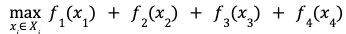where f1, f2, f3, f4 are latent functions that model metrics for each cohort. The main benefit here is instead of modeling a single global metric that is a function of a four-dimensional hyperparameter, we can model four metrics where each metric is a function of a one-dimensional hyperparameter. We hope to share more on this in a later post.

• Instead of just using online metrics, we can apply a multitask Bayesian optimization approach that combines observations from online A/B tests with a simple offline metrics simulator. This can help us improve modeling for metrics that have high variance.

## Acknowledgements

We would like to thank Jun JiaHaichao WeiHuiji GaoZheng LiAjith MuralidharanJane YuMohsen JamaliShipeng YuHema Raghavan, and Romer Rosales for their instrumental support, and Suman SundareshRupesh Gupta, and Matthew Walker for helping us improve the quality of this post. Finally, we are grateful to our fellow team members from AI Algorithms Foundation, Notifications AI, Feed AI, PYMK AI, and Ads AI teams for the great collaboration.